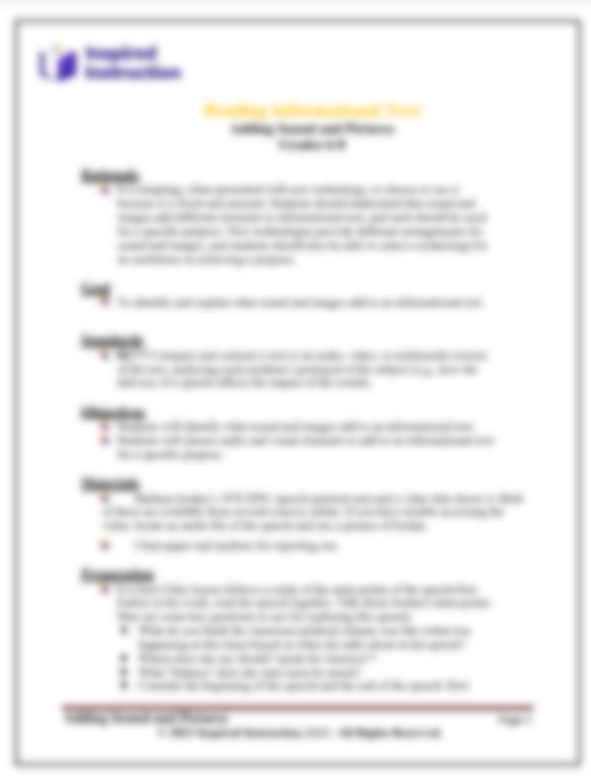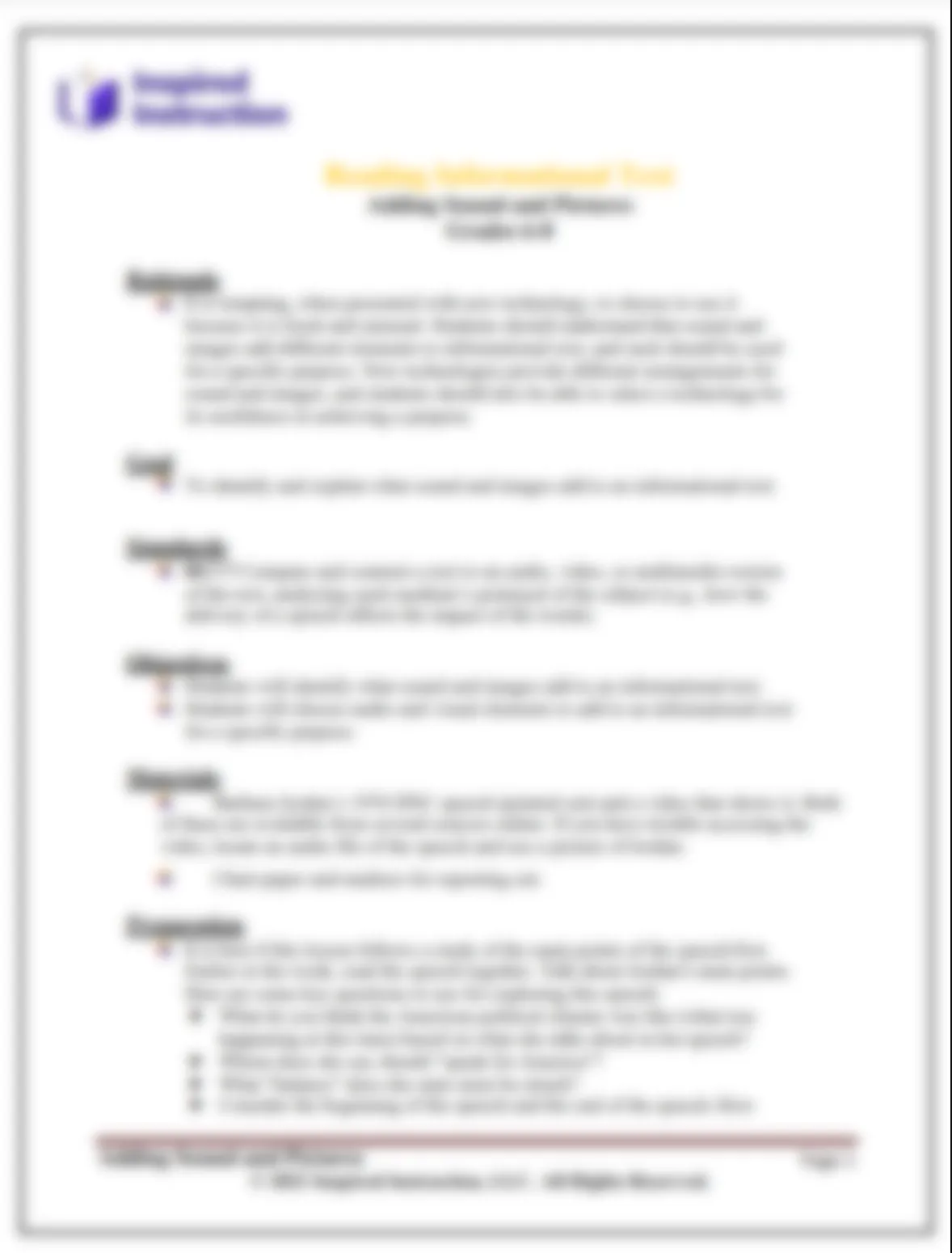# NJSLA Algebra II, Lesson 4: Mathematical Practices

The Standards for Mathematical Practice describe the habits of mind of an excellent math student and describe ways in which students should engage in mathematics as they grow in mathematical maturity and expertise throughout the elementary, middle and high school years.

The Standards for Mathematical Content are a balanced combination of procedure and understanding. Students who lack understanding of a topic may rely on procedures too heavily. Without a flexible base from which to work, they may be less likely to consider analogous problems, represent problems coherently, justify conclusions, and apply the mathematics to practical situations. They may have difficulty explaining the mathematics accurately to other students. The Standards for Mathematical Practice are designed to provide students with a flexible base to work from and to help students develop the ability to step back for an overview or to change course from a known procedure to find a shortcut.

The goal of all instruction in mathematics should be to find the points of intersection between the Standards for Mathematical Content and the Standards for Mathematical Practice. The content standards which set an expectation of understanding are potential "points of intersection" between the Standards for Mathematical Content and the Standards for Mathematical Practice.

Subject:
PARCC/NJSLA
Content Type:
Lesson Plans

NJSLA Algebra II, Lesson 4: Mathematical Practices

NJSLA Algebra II, Lesson 4: Mathematical Practices

NJSLA Algebra II, Lesson 4: Mathematical Practices

NJSLA Algebra II, Lesson 4: Mathematical Practices

NJSLA Algebra II, Lesson 4: Mathematical Practices

## This content is exclusive to Members## This content is exclusive to Partner members## This content is exclusive to Partner+ membersNJSLA Algebra II, Lesson 4: Mathematical PracticesSchoolDistrictOtherOops! Something went wrong while submitting the form.

NJSLA

### Aiming to Exceed Expectations: Strategies for Success on the NJSLA

NJSLA Mathematics

### Integrating NJSLA into Daily Instruction for Math

PARCC/NJSLA